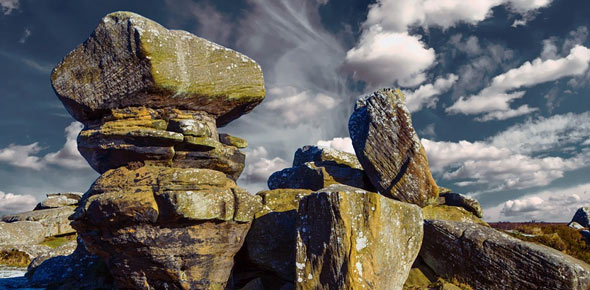# Geology 103: Oceanography Quiz

35 Questions | Total Attempts: 76SettingsRelated Topics
• 1.
A ________ is a large, fast moving ocean wave generated by an earthquake or submarine landslide.
• A.

Sieche

• B.

Swell

• C.

Tidal wave

• D.

Standing wave

• E.

Tsunami

• 2.
There is good evidence that the last big Cascadia earthquake to trigger a major tsunami occurred in
• A.

• B.

• C.

• D.

• 3.
If the earth were completely covered by water, in addition to a tidal bulge forming on the side of the earth nearest the Moon, another bulge forms on the opposite side of the earth due to:
• A.

The attraction by the sun

• B.

Refraction of the tidal wave

• C.

The Coriolis force

• D.

The centrifugal force

• 4.
The Autonomous Underwater Vehicle (AUV) described by Dr. Kipp Shearman
• A.

Was deployed in the open ocean for as much as three weeks at a time

• B.

Glided through the water column by changing its overall density

• C.

Measured dissolved oxygen, temperature and salinity

• D.

All of the above

• 5.
Plunging breaker usually form where there is a _______ bottom slope
• A.

Steep

• B.

Gentle

• 6.
Swell” is the term for waves:
• A.

That are still in the area of generation

• B.

That are generated by earthquakes rather than by the wind

• C.

That are generated by winds, but have moved out of the storm area and become regularly spaced as they cross the ocean

• D.

That have broken on the beach and are characterized by white water foam

• 7.
The distance over which the wind blows to create waves it termed:
• A.

Fetch

• B.

Distortion

• C.

Convection

• D.

Diffraction

• E.

Refraction

• 8.
When a wave crest is observed to move at 25 meters per second across the ocean, what is actually moving at 25 meters per second?
• A.

Ocean water

• B.

Wave height

• C.

Ocean salinity

• D.

Wave energy

• E.

Ocean currents

• 9.
Different wavelengths travel at different speeds according to the process called:
• A.

Dispersion

• B.

Refraction

• C.

Diffraction

• D.

Reflection

• E.

Declination

• 10.
Which of the following would help predict when and where waves will become unstable and break?
• A.

Wavelength

• B.

Wave height

• C.

Wave steepness

• D.

Wave prolongation

• E.

Wave dispersion

• 11.
The most abundant elements dissolved in seawater are:
• A.

Sodium (Na) and Chlorine (Cl)

• B.

Magnesium (mg) and mercury (hg)

• C.

Iron (fe) and silicon (si)

• D.

Calcium (ca) and sodium (na)

• E.

Uranium (U) and Plutonium (pu)

• 12.
The process in which water molecules change to a gas at temperatures below the boiling point is:
• A.

Transpiration

• B.

Sublimation

• C.

Deposition

• D.

Condensation

• E.

Evaporation

• 13.
Water’s unexpectedly high boiling and melting temperature depend on the _______ of the water molecule.
• A.

Light absorption

• B.

Density

• C.

Polar structure

• D.

Positive charge

• 14.
The average salinity of seawater is ____ parts per thousand (o/oo)
• A.

.35

• B.

3.5

• C.

35

• D.

350

• E.

3500

• 15.
A sharp increase in density with depth is called a/an
• A.

Pressure ridge

• B.

Pycnocline

• C.

Thermocline

• D.

Halocline

• E.

Isobar

• 16.
The average length of time an ion remains in solution in the ocean is termed:
• A.

Residence time

• B.

• C.

Half-life

• D.

Terminal time

• E.

A constant for all ions

• 17.
Seawater with a salinity of 35 o/oo freezes _____degrees C.
• A.

100

• B.

32

• C.

-1.9

• D.

0

• E.

98.7

• 18.
A/an _____ is a depth interval in the ocean where the temperature changes rapidly
• A.

Isotherm

• B.

Pycnocline

• C.

Thermocline

• D.

Halocline

• E.

Isobar

• 19.
The total dissolved materials in seawater is termed:
• A.

Density

• B.

Specific gravity

• C.

Toxicity

• D.

Salinity

• E.

Solubility

• 20.
The SOFAR channel is:
• A.

A submarine canyon cut by turbidites

• B.

A region of focusing of sound due to increasing pressure and decreasing temperature with the depth in the ocean

• C.

An eastern boundary current off Peru

• D.

A satellite broadcast frequency used for weather forecasting

• 21.
Over the ocean, ______ exceeds _____.
• A.

Evaporation; precipitation

• B.

Precipitation; evaporation

• C.

Wind; bathymetry

• D.

Temperature; salinity

• 22.
The well-lighted portion of the ocean is termed the _____ zone:
• A.

Aphotic

• B.

Dysphotic

• C.

Abyssal

• D.

Benthic

• E.

Photic

• 23.
High tides are highest during:
• A.

Spring tides

• B.

Neap tides

• C.

Mixed tides

• D.

Spring break

• 24.
Wind is caused by:
• A.

The phases of the moon

• B.

Solar flares

• C.

Differences in air pressure

• D.

The tides

• E.

Evaporation

• 25.
The Coriolis deflection is cause by:
• A.

The phases of the moon

• B.

The gravitational attraction of the sun

• C.

The combined gravitational attraction of the sun and moon

• D.

Apparent motion of object in the reference frame of the rotation earth

• E.

Atmospheric pressure